# Rectifiable curve

(diff) ← Older revision | Latest revision (diff) | Newer revision → (diff)

A curve having a finite length (cf. Line (curve)). Letbe a continuous parametric curve in three-dimensional Euclidean space, that is,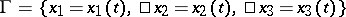,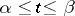, where the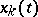,, are continuous functions on the interval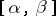. Let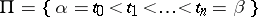be a partition ofand letbe the sequence of points oncorresponding to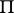. Also, let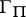be the polygonal arc inscribed inhaving vertices at. The length of this arc is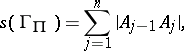where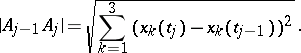Thenis called the length of the curve.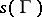does not depend on the parametrization of. If, thenis called a rectifiable curve. A rectifiable curvehas a tangent at almost every point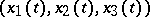, i.e. for almost all parameter values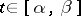. The study of rectifiable curves was initiated by L. Scheeffer  and continued by C. Jordan , who proved thatis rectifiable if and only if the functions,, are of bounded variation on(cf. Function of bounded variation).